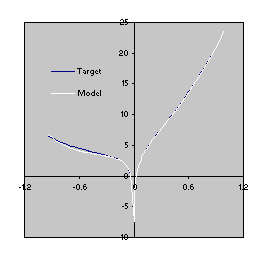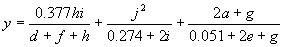Home
News
Author
Q&A
Tutorials
GEP Biblio
Contacts

Visit Gepsoft

Gene Expression Programming: Mathematical Modeling by an Artificial Intelligence

Direct manipulation of numerical constants

To solve the sequence induction problem using the facility to manipulate random constants, the set of functions F = {+, -, *} and the set of terminals T = {a, ?}. Furthermore, a set of integer random constants represented by the numerals 0-9 was used, that is, R = {0, 1, 2, 3, 4, 5, 6, 7, 8, 9}. The ephemeral random constant “?” ranged over the integers 0, 1, 2, and 3. The parameters used per run are shown in the first column of Table 4.7.

The perfect solution shown below was discovered on generation 99 of run 0 (the sub-ETs are linked by addition):

 Gene 0: ***a+*aaaa?a?4044044         A0 = {0, 1, 3, 0, 2, 0, 1, 1, 0, 1}     Gene 1: **+*a?a?aa???3858227         A1 = {3, 2, 2, 1, 3, 1, 1, 0, 3, 2}     Gene 2: /?a-?-a????aa5011303         A2 = {3, 2, 0, 0, 1, 0, 2, 1, 3, 1}     Gene 3: -?-*-aa??a?aa5485938         A3 = {3, 0, 1, 2, 0, 3, 3, 1, 3, 3}     Gene 4: a**/*aaa?aaaa2416267 A4 = {0, 2, 0, 2, 2, 2, 1, 0, 3, 3} (4.11)

As its expression shows, this program matches exactly the target sequence (4.9).

As shown in Table 4.7, the probability of success for this problem is 24%, considerably lower than the 98% obtained using the second approach. Surprisingly, the inclusion of numerical constants in the evolutionary toolkit results in worse performance. Thus, evolutionary algorithms find the numerical constants more easily if no assumptions whatsoever are a priori made. It is worth pointing out that only the prior knowledge of the solution enabled us, in this case, to choose correctly the type and the range of the random constants.

To find the “V” shaped function by explicitly using random constants F = {+, -, *, /, L, E, K, ~, S, C} (“L” represents the natural logarithm, “E” represents ex, “K” represents the logarithm of base 10, “~” represents 10x, “S” represents the sine function, and “C” represents the cosine) and T = {a, ?}. The set of rational random constants R = {0, 1, 2, 3, 4, 5, 6, 7, 8, 9}, and “?” ranged over the interval [-1, 1]. The parameters used per run are shown in the first column of Table 4.9. In the best run of this experiment, the best solution was found in generation 3631. Its genome is shown below (the sub-ETs are linked by addition):

 Gene 0: ++?~a*a???a?a0643987         A0 = {0.03, 0.019, 0.419, 0.247, 0.367, 0.689, 0.648, 0.245, 0.252, 0.904}     Gene 1: ~S/aSE?a?aaaa5670520         A1 = {0.476, 0.477, 0.786, 0.196, 0.92, 0.344, 0.934, 0.198, 0.107, 0.575}     Gene 2: ~CCLE*aa?a??a1654524         A2 = {0.298, 0.083, 0.466, 0.404, 0.912, 0.782, 0.392, 0.223, 0.548, 0.43}     Gene 3: /LE/-*?aaaaa?4324454         A3 = {0.947, 0.357, 0.549, 0.338, 0.465, 0.435, 0.837, 0.776, 0.602, 0.593}     Gene 4: ~*aCaE???aaa?8125208 A4 = {0.189, 0.357, 0.494, 0.38, 0.465, 0.88, 0.995, 0.971, 0.966, 0.294} (4.12a)

It has a fitness of 1978.177 and an R-square of 0.999914 evaluated over the set of 20 fitness cases and an R-square of 0.999216 evaluated against a testing set of 100 random points also chosen from the interval [-1, 1]. More formally, it corresponds to the following C++ function:

 double APSCfunction(double d[ ])      {           double dblTemp = 0;           dblTemp += ((pow(10,(d*0.648))+d)+0.03);           dblTemp += pow(10,sin((d/sin(exp(0.344)))));           dblTemp += pow(10,cos(cos(log(exp((d*d))))));           dblTemp += (log(((d*d)/0.465))/exp((d-d)));           dblTemp += pow(10,(d*cos(d)));           return dblTemp; } (4.12b)

where d0 corresponds to the independent variable.

This model is a very good approximation to the target function (4.10) as both the R-square and the comparison of the plots for the target function and the model show (Figure 4.7).Figure 4.7. Comparison of the target function (4.10) with the model (4.12) evolved by GEP with the facility to manipulate random constants. The R-square was evaluated over a testing set of 100 random points and is equal to 0.999216.

And, finally, for predicting sunspots using the facility for the manipulation of random numerical constants F = {+, -, *, /} and T = {a, b, c, d, e, f, g, h, i, j, ?}, which correspond, respectively, to t-10, t-9, ..., t-1. The set of rational random constants R = {0, 1, 2, 3, 4, 5, 6, 7, 8, 9}, and “?” ranged over the interval [-1, 1]. The parameters used per run are shown in the first column of Table 4.10. The best solution, found in run 90 after 4945 generations, is shown below (the sub-ETs are linked by addition):

 Gene 0: /*+i*h+?hfddh?c29898626         A0 = {0.387, 0.375, 0.373, 0.606, 0.363, 0.958, 0.211, 0.062, 0.12, 0.239}     Gene 1: *j/j++ii?afc?ad03960507         A1 = {0.274, 0.472, 0.153, 0.38, 0.887, 0.828, 0.378, 0.779, 0.414, 0.065}     Gene 2: /+++a++agee?gjd89794029 A2 = {0.433, 0.503, 0.333, 0.092, 0.066, 0.245, 0.592, 0.43, 0.051, 0.998} (4.13a)

It has a fitness of 86733.21 and an R-square of 0.84312712 evaluated over the set of 90 fitness cases. Mathematically, it corresponds to the following function:(4.13b)

It is worth noticing that, using this approach, the algorithm does in fact integrate constants in the evolved solutions, but the constants are, at least in some cases, very different from the expected ones (compare, for instance, the model of the “V” function with the function itself). Indeed, gene expression programming (and I believe, all evolutionary algorithms) can find the expected constants with a precision to the third or fourth decimal place if the target functions are simple polynomial functions with rational coefficients, otherwise a very creative solution will be found. For instance, the simple function below:

 y = 2.718a2 + 3.1416a (4.14)

can be exactly solved by GEP using the facility to manipulate random constants (see the performance and parameters in Table 4.11).

Table 4.11
Settings for the polynomial function with rational coefficients.

 Number of runs 100 Number of generations 1000 Population size 50 Number of fitness cases 10 (Table 4.12) Function set + - * / Terminal set a ? Random constants array length 10 Random constants range [-1,1] Head length 6 Number of genes 2 Linking function + Chromosome length 40 Mutation rate 0.044 One-point recombination rate 0.3 Two-point recombination rate 0.3 Gene recombination rate 0.1 IS transposition rate 0.1 IS elements length 1,2,3 RIS transposition rate 0.1 RIS elements length 1,2,3 Gene transposition rate 0.1 Random constants mutation rate 0.01 Dc specific transposition rate 0.1 Dc specific IS elements length 1,2,3 Selection range 25% Precision 0% Average best-of-run fitness 243.994 Average best-of-run R-square 0.999826

Table 4.12
Set of fitness cases for the polynomial function.

 a f(a) -8.5013 169.7278883 -0.8696 -0.676572453 3.7181 49.25514232 5.0878 86.34118911 -4.313 37.01043094 1.9775 16.84126999 -8.767 181.3638583 -5.5617 66.60191701 -1.4234 1.035098188 6.9014 151.1379353

Consider, for instance, the almost perfect solution below:

 Gene 0: *+++*+?aaa?a?4673929         A0 = {0.244, 0.966, 0.461, 0.762, 0.409, 0.567, 0.718, 0.746, 0.993, 0.236}     Gene 1: **??aa???a??a8610121 A1 = {0.103, 0.086, 0.038, 0.793, 0.307, 0.062, 0.051, 0.025, 0.046, 0.911} (4.15a)

It has an R-square equal to 0.999999999769 and, therefore, is an almost perfect match to the target function. Indeed, as its mathematical expression shows, the algorithm discovered the numerical constants of equation (4.14) with great precision:

 y = 2.718a2 + 3.14163a (4.15b)

Home | Contents | Previous | Next